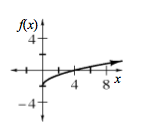### Home > INT3 > Chapter 1 > Lesson 1.1.2 > Problem1-22

1-22.

Make a complete graph of the function $f\left(x\right)=\sqrt{x}-2$. Fully describe the graph.

Make a table.

What is the smallest value of $x$ you can use?

Try using $0$, $1$, $2$, $3$, $4$, and $9$ as $x$-values. What happens when you try $−1$ or $−4$?

What is the smallest output value possible?